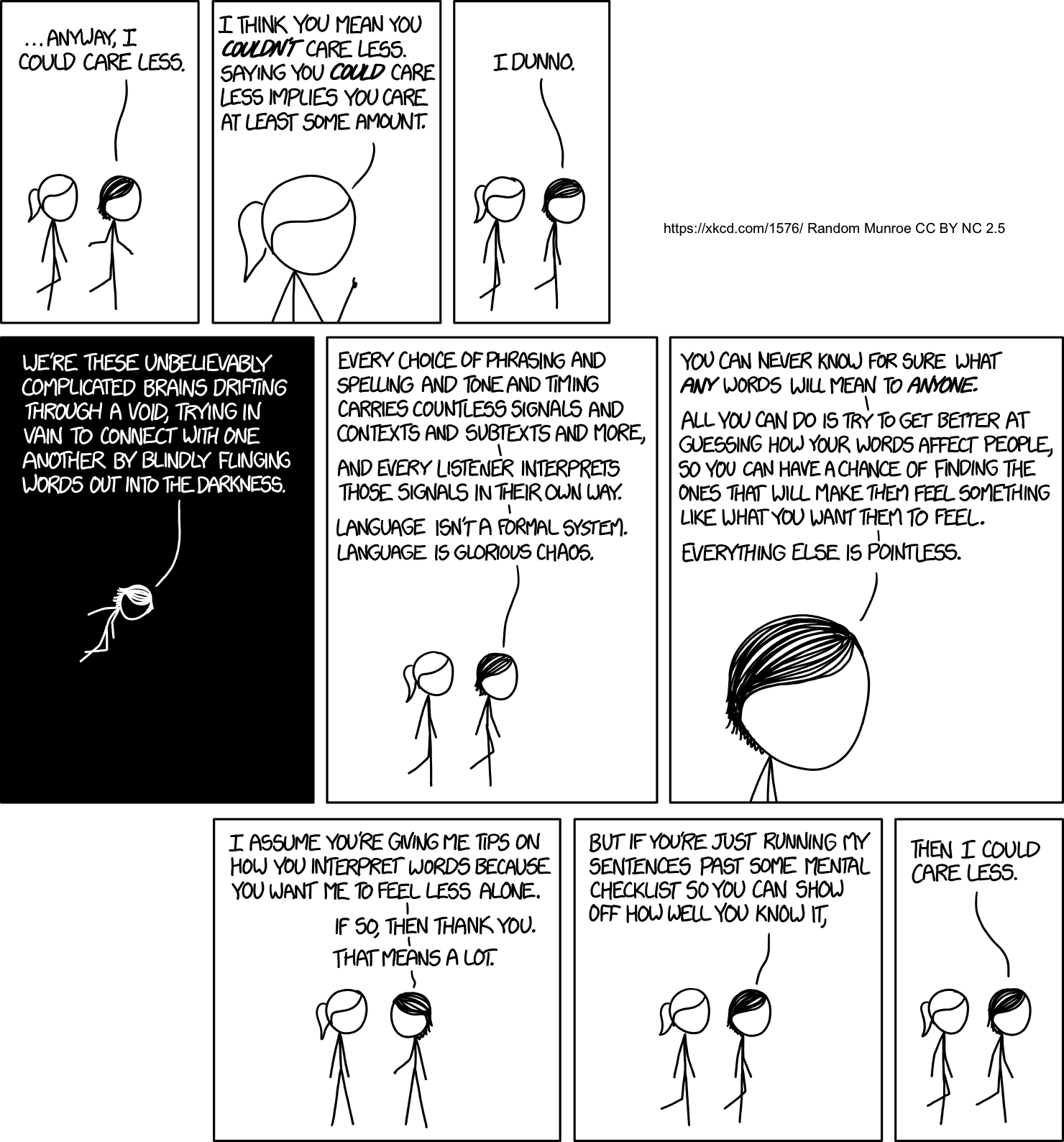# CS224N: NLP with Deep Learning | Winter2019 | Lecture1

May 30, 2020 · 4 mins read# Introduction and Word Vectors

• What is language?

Think about how complicated Human language is. - xkcd cartoon

Considering the information theory, Human language is so slow

Is the human language is just same with orangutan?

Commonest linguistic way of thinking of meaning is a denotational meaning(see referential linguistics)

• with computer

But this definition is hard to implement with computer

-> WordNet

But wordnet is incomplete because it can’t handle with

• Traditional approach (a localist representation)

treating each word as discrete symbols: As categorical variable

but with this approach, we can’t cover all of derivative.

Moreover, relationship can’t be represented using localist representation.

• Distributional Semantics

J. R. Firth 1957

Let’s see all of the examples of usage, and represent their appearance using dense vectors.

the picture is just projects of 100-d vectors to 2-d (will talk about this later) / Be sure to keep in mind when you see the word-embeddings, there’s original vector space.

Q. Are there standard features for i-th dimension in vector representation?

• Word2vec

Mikolov paper

vector representation is the only parameter with simple word2vec model(actually two) 1) center word vectors 2) context word vectors

• Objective function (changed little bit from likelihood)

1) Negative: minimize rather than maximize 2) divide by size of corpus(T) i.e. scaling: make it independent of corpus size 3) log: turned out works well when you do things like optimization - it changes the objective function, which was originally was product, to sum

• Prediction function

exp : makes number positive

this function has two parts

1) as doing the dot product, it calculates similarity (=relationship) between specific word(c) and context(o) 2) blue part means mapping from variable to probability distribution [^1]

Q->A: distributional of meaning assumes we have intelligent vector which knows what word will be, when I give other words. so, we get high probability

And notice a word has only one vector, regardless of the context. (since we have only one simple probability distribution)

(explained formula / how to calculate)

But be sure to you can calculate what’s happening in calculation process

we get this results, meaning we are sliding down the slope by 1) get a observed representation 2) 1

• jupyter

stanford has lightweight version of glove

disimilar could be useful, because this has multiple dimensions, so that each vector space has some feature, like women and man, and we can add/subtract that feature between word -> makes us be able to do analogy, using this semantic relationship.

and this is foundation of modern distributed neural representations of words

this is a fourth declensions noun.

So the plural of corpus is corpora.

And whereas if you say

core Pi everyone will know that you didn’t study Latin in high school.

[^1]

soft and max part

that’s sort of a hard max.

Um, soft- this is a softmax because the exponenti- you know,

if you sort of imagine this but- if we just ignore the problem

negative numbers for a moment and you got rid of the exp, um,

then you’d sort of coming out with

a probability distribution but by and large it’s so be fairly

flat and wouldn’t particularly pick out the max of

the different XI numbers whereas when you exponentiate them,

that sort of makes big numbers way bigger and so, this,

this softmax sort of mainly puts mass where the max’s or the couple of max’s are.

the context word and we’re subtracting from that what our model thinks um,

the context should look like.

What does the model think that the context should look like?

This part here is formal in expectation.

So, what you’re doing is you’re finding the weighted average

of the models of the representations of each word,

multiplied by the probability of it in the current model.

So, this is sort of the expected context word according to our current model,

and so we’re taking the difference between

the expected context word and the actual context word that showed up,

and that difference then turns out to exactly give

us the slope as to which direction we should be

walking changing the words

representation in order to improve our model’s ability to predict.

1. the observed representation of